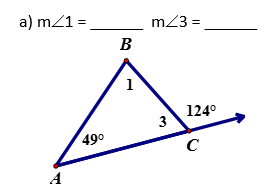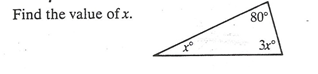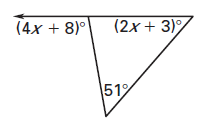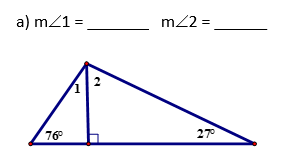#### MAT-HS.G-CO.10.b

 MAT-HS Targeted Standards(G) Concept: Geometry(CO) Domain: CongruenceCluster: Prove geometric theorems MAT-HS.G-CO.10.b Apply theorems about triangles. (Theorems include: measures of interior angles of a triangle sum to 180 degrees; base angles of isosceles triangles are congruent; the segment joining midpoints of two sides of a triangle is parallel to the third side and half the length; the medians of a triangle meet at a point. (Focus on Applying)

• I can
• I can

• I can
• I can

• I can
• I can

• I can
• I can

## Proficiency Scale

 Score Description Sample Activity Advanced In addition to Score 3.0, the student demonstrates in-depth inferences and applications regarding more complex material that go beyond end of instruction expectations. Analyze a solution and correct the errors Investigate and provide multiple methods for finding a solution to a given problemFind the measures of these angles in two different ways. Explain the steps you took for each method. 3.5 In addition to Score 3.0 performance, the student demonstrates in-depth inferences and applications regarding the more complex content with partial success. Proficient “The Standard.” The student demonstrates no major errors or omissions regarding any of the information and processes that were end of instruction expectations. Apply theorems (postulates, definitions, etc.) about triangles to solve problems Justify how to set up a problem using theorems (postulates, definitions, etc.) about triangles2.5 The student demonstrates no major errors or omissions regarding the simpler details and processes (Score 2.0 content) and partial knowledge of the more complex ideas and processes (Score 3.0 content). Progressing The student demonstrates no major errors or omissions regarding the simpler details and processes but exhibits major errors or omissions regarding the more complex ideas and processes (Score 3.0 content).   Identify or relate the theorem used to set up the problem Define the vocabulary associated with triangle theorems Can identify the components of triangles - 1.5 The student demonstrates partial knowledge of the simpler details and processes (Score 2.0 content) but exhibits major errors or omissions regarding the more complex ideas and procedures (Score 3.0 content). Beginning With help, the student demonstrates a partial understanding of some of the simpler details and processes (Score 2.0 content) and some of the more complex ideas and processes (Score 3.0 content). - 0.5 With help, the student demonstrates a partial understanding of some of the simpler details and processes (Score 2.0 content) but not the more complex ideas and processes (Score 3.0 content). 0.0 Even with help, the student demonstrates no understanding or skill. -

## Resources

 Web Vocab